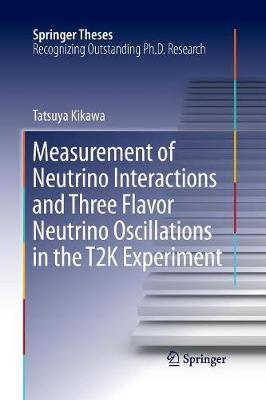•Measurement of Neutrino Interactions and Three Flavor Neutrino Oscillations in the T2K Experiment - Springer Theses (Paperback)

(author)
£74.99
Paperback 264 Pages / Published: 23/08/2016
• We can order this

Usually dispatched within 3 weeks

This book is based on the author's work in the T2K long-baseline neutrino oscillation experiment, in which neutrinos are generated by a proton beam and are detected by near and far neutrino detectors. In order to achieve the precise measurement of the neutrino oscillation, an accurate understanding of the neutrino beam and the neutrino interaction is essential. Thus, the author measured the neutrino beam properties and the neutrino interaction cross sections using a near neutrino detector called INGRID and promoted a better understanding of them. Then, the author performed a neutrino oscillation analysis using the neutrino beam and neutrino interaction models verified by the INGRID measurements. As a result, some values of the neutrino CP phase are disfavored at the 90% confidence level. If the measurement precision is further improved, we may be able to discover the finite CP phase which involves the CP violation. Thus, this result is an important step towards the discovery of CP violation in the lepton sector, which may be the key to understanding the origin of the matter-antimatter asymmetry in the universe.

Publisher: Springer Verlag, Singapore
ISBN: 9789811012983
Number of pages: 264
Weight: 4336 g
Dimensions: 235 x 155 mm
Edition: Softcover reprint of the original 1st ed. 201